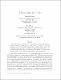## Toida's conjecture is true2002
Dobson, Edward
Morris, Joy
##### Publisher
Electronic Journal of Combinatorics
##### Abstract
Let S be a subset of the units in Zn. Let Γ be a circulant graph of order n (a Cayley graph of Zn) such that if ij ∈ E(Γ), then i − j (mod n) ∈ S. Toida conjectured that if Γ0 is another circulant graph of order n, then Γ and Γ 0 are isomorphic if and only if they are isomorphic by a group automorphism of Zn. In this paper, we prove that Toida’s conjecture is true. We further prove that Toida’s conjecture implies Zibin’s conjecture, a generalization of Toida’s conjecture.
##### Description
Sherpa Romeo green journal: open access
##### Keywords
Graph , Isomorphic , Automorphism , Toida's conjecture , Zibin's conjecture , Caley , Digraphs
##### Citation
Dobson, E., & Morris, J. (2002). Toida's conjecture is true. Electronic Journal of Combinatorics, 9(1), R35